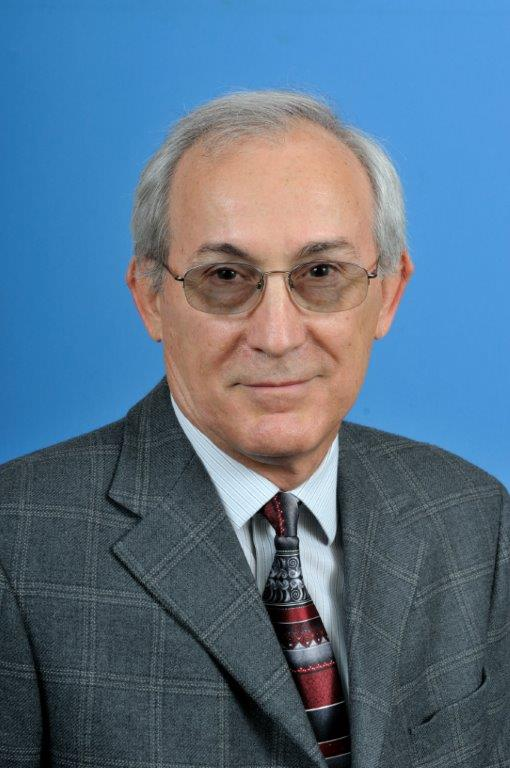# Dr. Vassil YorgovProfessor in Mathematics

Office: Science & Technology 220

Phone: (910) 672-1675

Email: vyorgov@uncfsu.edu

Personal Homepage: http://faculty.uncfsu.edu/vyorgov/

BIOGRAPHY

Dr. Yorgov is a professor of mathematics at the Department of Mathematics and Computer Science at FSU since 2006. He joined the Department at 2002 as associate professor.  His research is in the area of error-correcting codes and combinatorics. He has 29 scholarly publications which are cited in 372 works of other researchers.

TEACHING

MATH 121 Introduction to College Algebra
MATH 123 College Algebra
MATH 129 Precalculus Mathematics I
MATH 130 Precalculus Mathematics II
MATH 140 Applied Calculus
MATH 150 Discrete Mathematics I
MATH 361 Intro Mod Algebra I
MATH 362 Intro Mod Algebra II
MATH 507 Linear Algebra 1
MATH 511 Abstract Algebra I
MATH 611 Linear Algebra II
MATH 612, Abstract Algebra II

RESEARCH INTERESTS

Automorphisms of Linear Codes
Extremal Self-Dual Codes
Weight Distribution of Linear Codes
Codes over Rings
Weighing Matrices

SELECTED PUBLICATIONS

Yorgov, V., Yorgov, D. (2014). The Automorphism Group of a Self-Dual [72,36,16] Code Does Not Contain Z4. IEEE Trans. Inform. Theory, 60(6), 3302 - 3307.
Yorgov, V. (2013). On the Existence of Certain Circulant Weighing Matrices. Journal of Combinatorial Mathematics and Combinatorial Computing, 86(4), 73-86.
Mitchell, K., Yorgov, V. (2011). A Search for New Optimal Singly-Even Self-Dual Codes of Length 48. Explorations, VI 2011, 123-132. www.uncw.edu/csurf/explorations.html
Yorgov, V. (2011). Erratum to "The extremal codes of length 42 with automorphism of order 7" [Discrete Math. 190 (1998) 201-213]. Discrete Mathematics, 311(16), 1860-1861.
Yorgov, V. (2006). On the Automorphism Group of a Putative Code. IEEE Transactions on Information Theory, 52(4), 1724-1726.